mraa  2.0.0 Low Level Skeleton Library for Communication on GNU/Linux platforms
Gpio Class Reference

## Detailed Description

This file defines the gpio interface for libmraa

/* initialize GPIO pin */
mraa::Gpio gpio_1(GPIO_PIN_1);
/* initialize GPIO pin */
mraa::Gpio gpio_2(GPIO_PIN_2);
/* set GPIO to output */
status = gpio_1.dir(mraa::DIR_OUT);
if (status != mraa::SUCCESS) {
printError(status);
return EXIT_FAILURE;
}
/* set gpio 24 to output */
status = gpio_2.dir(mraa::DIR_OUT);
if (status != mraa::SUCCESS) {
printError(status);
return EXIT_FAILURE;
}
/* toggle both GPIO's */
while (flag) {
status = gpio_1.write(1);
if (status != mraa::SUCCESS) {
printError(status);
return EXIT_FAILURE;
}
status = gpio_2.write(0);
if (status != mraa::SUCCESS) {
printError(status);
return EXIT_FAILURE;
}
sleep(1);
status = gpio_1.write(0);
if (status != mraa::SUCCESS) {
printError(status);
return EXIT_FAILURE;
}
status = gpio_2.write(1);
if (status != mraa::SUCCESS) {
printError(status);
return EXIT_FAILURE;
}
sleep(1);
}

## Public Member Functions

Gpio (int pin, bool owner=true, bool raw=false)

Gpio (void *gpio_context)

~Gpio ()

Result edge (Edge mode)

Result isr (Edge mode, void(*fptr)(void *), void *args)

Result isrExit ()

Result mode (Mode mode)

Result dir (Dir dir)

Result write (int value)

Result useMmap (bool enable)

int getPin (bool raw=false)

Result inputMode (InputMode mode)

Result outputMode (OutputMode mode)

## Constructor & Destructor Documentation

 Gpio ( int pin, bool owner = `true`, bool raw = `false` )
inline

Instantiates a Gpio object

Parameters
 pin pin number to use owner (optional) Set pin owner, default behaviour is to 'own' the pin if we exported it. This means we will close it on destruct. Otherwise it will get left open. This is only valid in sysfs use cases raw (optional) Raw pins will use gpiolibs pin numbering from the kernel module. Note that you will not get any muxers set up for you so this may not always work as expected.

Here is the call graph for this function:Gpio ( void * gpio_context )
inline

Gpio Constructor, takes a pointer to the GPIO context and initialises the GPIO class

Parameters
 gpio_context void * to GPIO context
 ~Gpio ( )
inline

Gpio object destructor, this will only unexport the gpio if we where the owner

Here is the call graph for this function: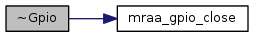## Member Function Documentation

 Result edge ( Edge mode )
inline

Set the edge mode for ISR

Parameters
 mode The edge mode to set
Returns
Result of operation

Here is the call graph for this function:Result isr ( Edge mode, void(*)(void *) fptr, void * args )
inline

Sets a callback to be called when pin value changes

Parameters
 mode The edge mode to set fptr Function pointer to function to be called when interrupt is triggered args Arguments passed to the interrupt handler (fptr)
Returns
Result of operation

Here is the call graph for this function: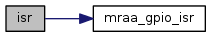Here is the caller graph for this function: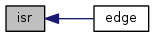Result isrExit ( )
inline

Exits callback - this call will not kill the isr thread immediately but only when it is out of it's critical section

Returns
Result of operation

Here is the call graph for this function: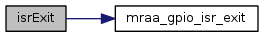Result mode ( Mode mode )
inline

Change Gpio mode

Parameters
 mode The mode to change the gpio into
Returns
Result of operation

Here is the call graph for this function:Here is the caller graph for this function:Result dir ( Dir dir )
inline

Change Gpio direction

Parameters
 dir The direction to change the gpio into
Returns
Result of operation

Here is the call graph for this function: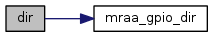Here is the caller graph for this function:Dir readDir ( )
inline

Exceptions
 std::runtime_error in case of failure
Returns
Result of operation

Here is the call graph for this function: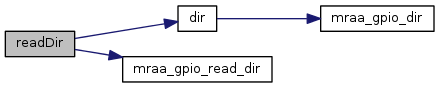int read ( )
inline

Read value from Gpio

Returns
Gpio value

Here is the call graph for this function: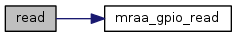Result write ( int value )
inline

Write value to Gpio

Parameters
 value Value to write to Gpio
Returns
Result of operation

Here is the call graph for this function: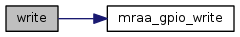Result useMmap ( bool enable )
inline

Enable use of mmap i/o if available.

Parameters
 enable true to use mmap
Returns
Result of operation

Here is the call graph for this function:int getPin ( bool raw = `false` )
inline

Get pin number of Gpio. If raw param is True will return the number as used within sysfs. Invalid will return -1.

Parameters
 raw (optional) get the raw gpio number.
Returns
Pin number

Here is the call graph for this function: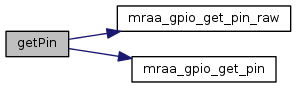Result inputMode ( InputMode mode )
inline

Change Gpio input mode

Parameters
 mode The mode to change the gpio input
Returns
Result of operation

Here is the call graph for this function:Result outputMode ( OutputMode mode )
inline

Change Gpio output driver mode

Parameters
 mode Set output driver mode
Returns
Result of operation

Here is the call graph for this function: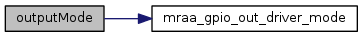The documentation for this class was generated from the following file: